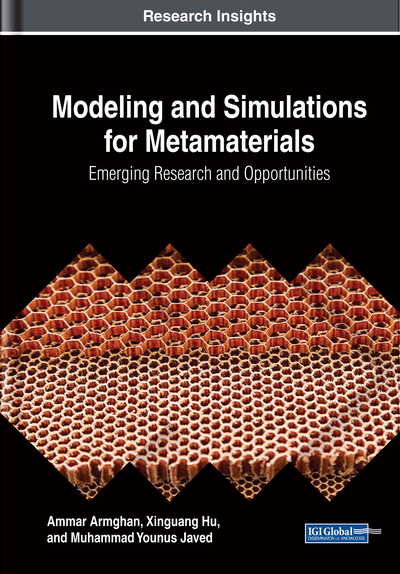# Homogenization and Parameter Retrieval

DOI: 10.4018/978-1-5225-4180-6.ch005
Available
\$37.50
No Current Special Offers

## Abstract

This chapter describes the metamaterials based on periodic structures inhabiting theoretically a distinctive place among effective media and photonic crystals. The S-parameter retrieval procedures that have been exploited recently to describe metamaterials have been made known to be effective for metamaterials having asymmetric unit cells. The single unit cell of a conventional symmetric (in the propagation direction) metamaterial arrangement is illustrated, and it produces negative permittivity and permeability.
Chapter Preview
Top

## 5.1 Introduction

Structure of metamaterial and mixture of the photonic crystal as shown in the Figure 1, can model or measures the scattering from that, hit it with the incident wave from the left it reflects or transmit likewise if hit it from the right it transmits or backward reflect that is why it called the scattering parameters, can either simulate them or model them. Now with the second material that is homogenous so it is a smooth continuous material with some set of electromagnetic properties, and for the same thickness if simulate that and get same scattering parameters as discussed earlier these would be the effective electromagnetic properties of the metamaterial (Bossavit, 1995). So it is one for one replacement of your engineered material with homogeneous properties called the effective properties.

So let’s consider the slab of the metamaterial as shown in Figure 2, a finite difference frequency domain simulation of a subwavelength grating, so look at the cross-section of the gratings a one tooth with the dielectric constant of 6.0 and has an air gap between the teeth and there is an air on the top and the bottom. So the question is give the slab of thickness d what are the effective material properties of that, if do that correctly, should be able to replace that grating with the homogeneous slab with the effective dielectric constant same thickness and the same transmission and reflection can be seen. Now, consider the set of data, simulate the structure one time and calculated whatever data of transmission and reflection get from the slab of metamaterial that would be Rmeta and Tmeta as shown in equation 1, so that is the transmission and reflection of that metamaterial grating (Guerin, Labeyrie, & Guillon, 1995). Then make some guesses what would be the dielectric constant since making this with the dielectric constant either 1 or 6. As it is already known that it would be somewhere around greater than 1.0 or less than 6.0. So, set a sweep of effecting electromagnetic parameters between 1 and 6 as shown in Figure 2.

So then pick up the dielectric constant and calculate the transmission and reflection using the technique like transfer matrix method and given those Rhomo and Thomo calculated an overall by using equation 1, so as shown in Figure 2 increasing at the same time both the thickness of the gratings and thickness of the slab, so that is both the same and for each horizontal thickness sweep all the possible dielectric constant and calculated the error. So for dielectric constant and thickness of the slab. In principle as changing the thickness if the slab of the metamaterial does act like an effective material that should not change its effective properties. So the effective dielectric constant region is somewhere between 3.0-3.5 as it can be seen from Figure 2. But notice that some other minimum regions as well, as it goes thicker and thicker gratings there is more and more of these possible answers, and in fact, if it is just fixed to one slab thickness, there would be multiple correct answers, so how can someone determine which one is the correct answer. So when there are multiple correct solutions that are called branching. That is why it is too complicated because have to sort out which one is the correct answer.

(5.1)

## Complete Chapter List

Search this Book:
Reset